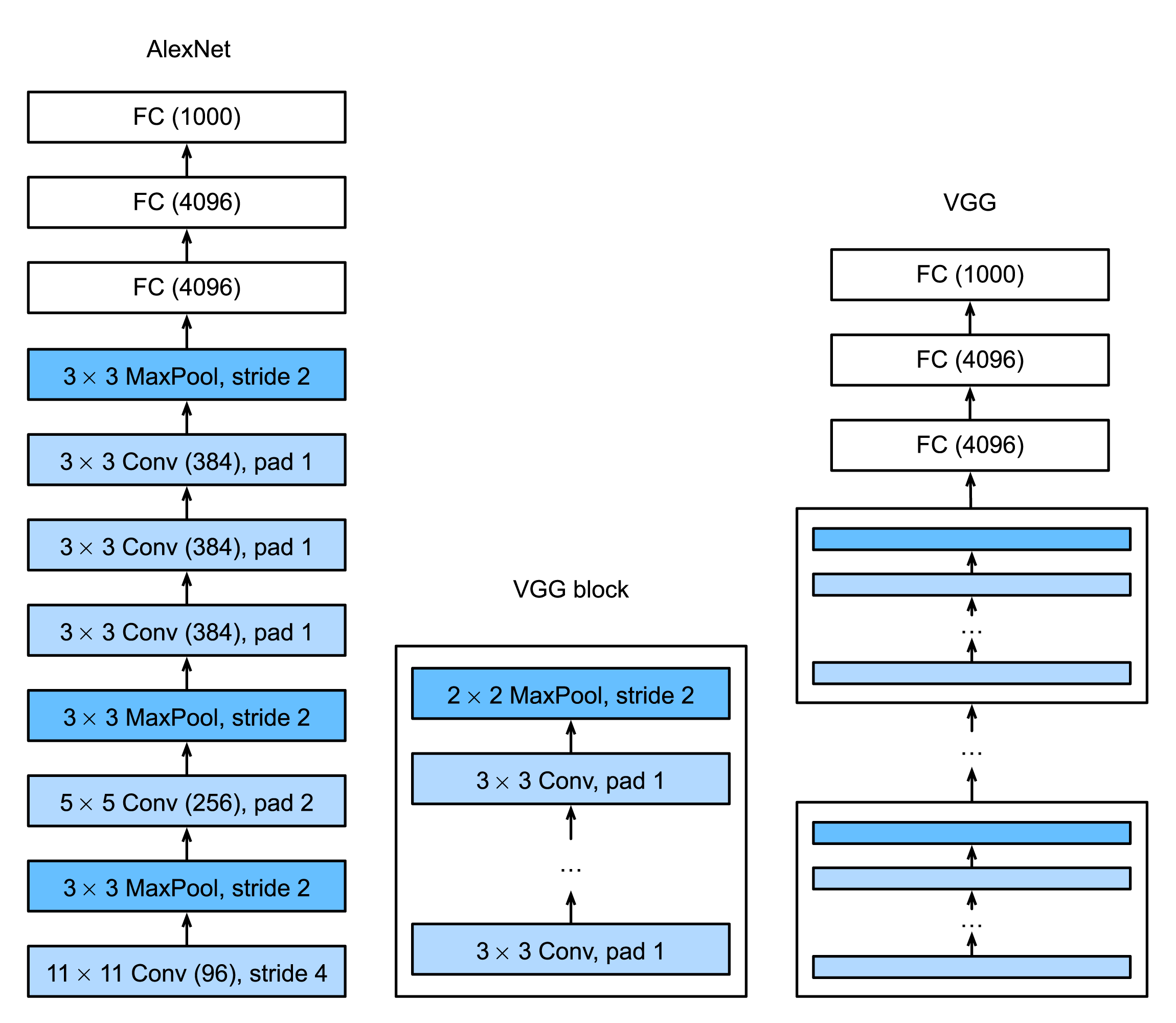# VGG：使用基础卷积块的网络

AlexNetLeNet的基础上增加了卷积层，但AlexNet对卷积窗口、输出通道数和构造顺序均做了大量的调整。虽然AlexNet指明了深度卷积神经网络有出色的表现，但并没有提供简单的规则以指导后来的研究者如何设计新的网络。

VGG网络是Oxford的Visual Geometry Group组提出的，VGG网络是实验室名字的缩写。VGG论文阐述了其在ImageNet 2014挑战赛上的一些发现，提出了可以通过重复使用基础块（Block）来构造深度模型。

## VGG块

1. 一个卷积层
2. 一个非线性激活函数，例如ReLU
3. 一个池化层，例如最大池化层

VGG论文中提出的方法是：连续使用数个相同的填充为1、窗口形状为3 × 3的卷积层后紧接一个步幅为2、窗口形状为2 × 2​的最大池化层。卷积层保持输入和输出的高和宽不变，而池化层对输入的尺寸减半。

def vgg_block(num_convs, in_channels, out_channels):
'''
Returns a block of convolutional neural network

Parameters:
num_convs (int): number of convolutional layers this block has
in_channels (int): input channel number of this block
out_channels (int): output channel number of this block

Returns:
a nn.Sequential network:
'''
layers=[]
for _ in range(num_convs):
# (input_shape - 3 + 2 + 1) / 1 = input_shape
layers.append(nn.ReLU())
in_channels = out_channels
# (input_shape - 2 + 2) / 2 = input_shape / 2
layers.append(nn.MaxPool2d(kernel_size=2,stride=2))
return nn.Sequential(*layers)


## VGG网络

VGG与AlexNet、LeNet类似，VGG网络分为两大部分，前半部分是卷积层和池化层模块，后半部分是全连接层模块。从AlexNet到VGG：使用基础块构建深度神经网络

def vgg(conv_arch):
'''
Returns the VGG network

Parameters:
conv_arch ([(int, int)]): a list which contains vgg block info.
the first element is the number of convolution layers this block have.
the latter element is the output channel number of this block.

Returns:
a nn.Sequential network:
'''
# The convolutional part
conv_blks=[]
in_channels=1
for (num_convs, out_channels) in conv_arch:
conv_blks.append(vgg_block(num_convs, in_channels, out_channels))
in_channels = out_channels

return nn.Sequential(
*conv_blks, nn.Flatten(),
# The fully-connected part
nn.Linear(out_channels * 7 * 7, 4096), nn.ReLU(), nn.Dropout(0.5),
nn.Linear(4096, 4096), nn.ReLU(), nn.Dropout(0.5),
nn.Linear(4096, 10))


conv_arch = ((1, 64), (1, 128), (2, 256), (2, 512), (2, 512))

net = vgg(conv_arch)


## 小结

1. VGG通过多个基础卷积块来构造网络。基础块需要定义卷积层个数和输入输出通道数。
2. VGG论文认为，更小（例如3 × 3）的卷积层比更大的卷积层效率更高，因为可以增加更多的层，同时参数又不至于过多。

1. Simonyan, K., & Zisserman, A. (2014). Very deep convolutional networks for large-scale image recognition.
2. http://d2l.ai/chapter_convolutional-modern/vgg.html